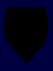STEWARDSHIP the assumption of responsibility for the welfare of the world
 SITE MAP REFERENCE BASE LOWEST COMMON DENOMINATOR   O.T. FORD   The expression lowest common denominator has been adopted into the vernacular to refer to an unsatisfactory compromise between two or more positions: legal protections have been reduced to the lowest common denominator. This is, however, not simply a misapplication of a mathematical analogy, but a logical error in basic English usage that is not dependent on mathematical knowledge. The expression should be greatest common factor. A denominator in math is the divisor of a fraction, the size of the parts the whole has been divided into  the bottom number in fractional notation. In the expression 3/4, 3 is the numerator and 4 is the denominator. The whole is divided into four equal parts, and we have three of them. The lowest common denominator (LCD) is a specific application of the least common multiple (LCM), which is the smallest number that is a whole-number multiple of two other whole numbers, the smallest number the two other numbers can be evenly divided into. The LCM of 4 and 6 is 12: 4 = 2 × 2, 6 = 2 × 3, the LCM must have at least two 2s (to be a multiple of 4) and at least a 2 and a 3 (to be a multiple of 6), thus is 2 × 2 × 3, or 12. The LCM is used for the LCD. In order to add the fractions 1/4 and 5/6, we need to know what denominator would allow us to convert both fractions to retain a whole numerator. 1/4 can be expressed as 3/12; 5/6 can be expressed as 10/12. 1/4 + 5/6 = 3/12 + 10/12 = 13/12 or 11/12. This mathematical term has been borrowed into common language because of what it seems to suggest, lowness; but it is a poor representation of the actual concept. When the speaker says that an element of a set has been reduced to the lowest common denominator, he in fact means that it has been reduced to the largest element which each member possesses in common  to use the correct mathematical analogy, the greatest common factor. The greatest common factor (GCF) is the largest (whole) number that is present as a factor in each of two or more numbers, the largest number that can be evenly divided into each. The greatest common factor of 24 and 30 is 6. 24 = 2 × 2 × 2 × 3, and 30 = 2 × 3 × 5. Each contains 2 × 3, or 6. What makes a greatest common factor so low is the requirement that it be in common. The layperson invoking the race to the bottom knows that the bottom is not zero. For example, in a free-trade negotiation between two states, one of them will often have weaker environmental protections or labor laws. While it may be politically useful to insinuate that the result of the negotiations will be a free trade area with no environmental protections or labor laws, the actual worst-case scenario is that environmental protections and labor laws will be harmonized to match those of the state with the weaker laws; that is, the result will be the best protections that both states have in common, the greatest common factor. It is true that, in the case of trade, a different process might follow, in which manufacturers free to move goods across the border choose to locate their plants in the state with the weaker protections and each state then competes to have the weaker protections, an actual race to the bottom. This does genuinely happen, as in the case of taxation, especially corporate tax rates. But the process of bidding protections down follows only if the protections are the subject of competition between rivals, rather than negotiation between partners as with free trade areas; and in any case, it has nothing to do with the lowest common denominator.   © O.T. FORD THE STEWARDSHIP Home of the Stewardship Projectand O.T. Ford